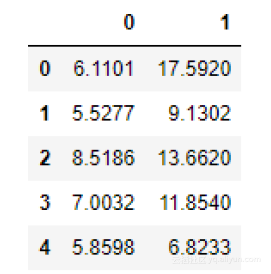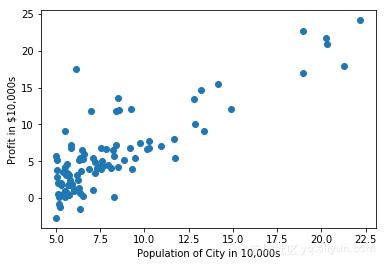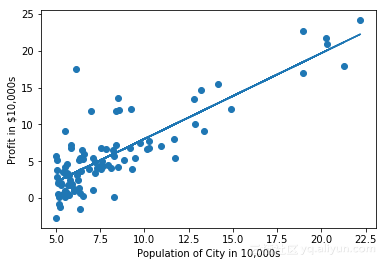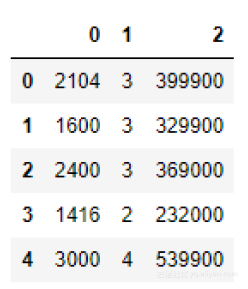# Andrew Ng经典机器学习课程的Python实现（第1部分）

+关注继续查看

1、这会帮助那些想要Python版本课程的人；

2.、对于有些R语言爱好者来说，他们也愿意学习熟悉的那些算法的Python实现，那会受益匪浅；

ex1data1.txt文件包含了我们线性回归练习的数据集。第一列表示城市的人口，第二列是该城市的食品货车的收益。如果收益为负则表示亏损。

importnumpy as np
import pandas as pd
import matplotlib.pyplot as plt

（在现实生活中我们遇到的许多问题都是多维的，不能仅仅用二维图来表示。要创建多维的表达方式，必须要灵活地运用各种表现形式，如色彩、形状、深度等。）

X = data.iloc[:,0] # read first column
y = data.iloc[:,1] # read second column
m = len(y) # number of training example
data.head() # view first few rows of the dataplt.scatter(X, y)
plt.xlabel('Population of City in 10,000s')
plt.ylabel('Profit in $10,000s') plt.show()添加拦截项 在下面的代码中，我们给数据添加另一个维度以适应拦截项（这么做的原因已在视频中进行了解释）。我们还将参数theta初始化为0，并把学习率alpha初始化为0.01 X = X[:,np.newaxis] y = y[:,np.newaxis] theta = np.zeros([2,1]) iterations = 1500 alpha = 0.01 ones = np.ones((m,1)) X = np.hstack((ones, X)) # adding the intercept term 使用np.newaxis可以将一维数组(shape: N elements)转换为行向量(shape: N rows, 1 column)或列向量(shape: 1 row, N columns)。在这里，我们将X和y重新排列到列向量里。 下一步，我们将计算成本和梯度下降，Andrew Ng在视频讲座中很好地讲解了这一操作过程。这里我仅提供Andrew Ng在讲座中使用的基于Python的伪代码。 成本计算 defcomputeCost(X, y, theta): temp = np.dot(X, theta) - y return np.sum(np.power(temp, 2)) / (2*m) J = computeCost(X, y, theta) print(J) 你应该期望看到成本的计算结果是32.07。 用梯度下降法求最优参数 defgradientDescent(X, y, theta, alpha, iterations): for _ in range(iterations): temp = np.dot(X, theta) - y temp = np.dot(X.T, temp) theta = theta - (alpha/m) * temp return theta theta = gradientDescent(X, y, theta, alpha, iterations) print(theta) 期望的theta的范围[-3.6303, 1.1664] 我们现在有了优化的theta值，利用上面的theta值来计算： J = computeCost(X, y, theta) print(J) 上面的输出应该会给你一个比32.07更好的结果：4.483。 绘制最佳拟合线图 plt.scatter(X[:,1], y) plt.xlabel('Population of City in 10,000s') plt.ylabel('Profit in$10,000s')
plt.plot(X[:,1], np.dot(X, theta))
plt.show()Import numpy as np
import pandas as pd

X = data.iloc[:,0:2] # read first two columns into X
y = data.iloc[:,2] # read the third column into y
m = len(y) # no. of training samples· 从数据集中减去每个特征的平均值；

· 减去平均值之后，再按各自的“标准偏差”缩放（分配）特征值；

X = (X - np.mean(X))/np.std(X)

ones = np.ones((m,1))
X = np.hstack((ones, X))
alpha = 0.01
num_iters = 400
theta = np.zeros((3,1))
y = y[:,np.newaxis]

defcomputeCostMulti(X, y, theta):
temp = np.dot(X, theta) - y
return np.sum(np.power(temp, 2)) / (2*m)
J = computeCostMulti(X, y, theta)
print(J)

m = len(y)
for _ in range(iterations):
temp = np.dot(X, theta) - y
temp = np.dot(X.T, temp)
theta = theta - (alpha/m) * temp
return theta
theta = gradientDescentMulti(X, y, theta, alpha, num_iters)
print(theta)

J = computeCostMulti(X, y, theta)
print(J)41 0Python聊天机器人实现代码【NLTK】

2202 0Andrew Ng深度学习课程笔记

2445 01Python全栈之路系列Web框架介绍
1242 0Andrew Ng机器学习课程笔记--week1（机器学习介绍及线性回归）

828 0Andrew Ng机器学习课程笔记--week4(神经网络)
Neural Networks: Representation 一、 内容概要 Neural Network Model Representation 1 Model Representation 2 Applications Examples and Intuitions 1 Examples and Intuitions 2 Multiclass Classification 二、重点&难点 1. Neural Network 1）Model Representation 1 首先需要明确一些符号的意思，以方便后面的阅读。
913 0Andrew Ng机器学习课程笔记--汇总

1283 0Andrew Ng机器学习课程笔记--week9(上)(异常检测&推荐系统)

903 0Andrew Ng机器学习课程笔记--week8(K-means&PCA)
Unsupervised Learning 本周我们讲学习非监督学习算法，会学习到如下概念 聚类（clustering） PCA(Principal Componets Analysis主成分分析)，用于加速学习算法，有时在可视化和帮助我们理解数据的时候会有难以置信的作用。
990 0Python 实现 Syslog 用于收集Cisco ASA Firewall 日志
5375 0
【方向】

706

5